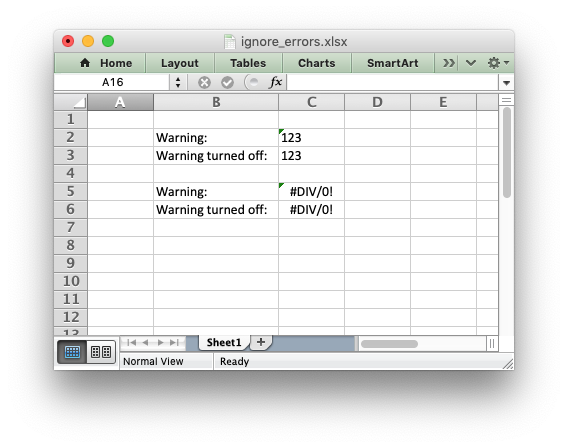libxlsxwriter
ignore_errors.c

Example of hiding worksheet errors and warnings./*
* An example of turning off worksheet cells errors/warnings using
* libxlsxwriter.
*
* Copyright 2014-2021, John McNamara, jmcnamara@cpan.org
*
*/
#include "xlsxwriter.h"
int main() {
lxw_workbook *workbook = workbook_new("ignore_errors.xlsx");
lxw_worksheet *worksheet = workbook_add_worksheet(workbook, NULL);
/* Write strings that looks like numbers. This will cause an Excel warning. */
worksheet_write_string(worksheet, CELL("C2"), "123", NULL);
worksheet_write_string(worksheet, CELL("C3"), "123", NULL);
/* Write a divide by zero formula. This will also cause an Excel warning. */
worksheet_write_formula(worksheet, CELL("C5"), "=1/0", NULL);
worksheet_write_formula(worksheet, CELL("C6"), "=1/0", NULL);
/* Turn off some of the warnings: */
/* Write some descriptions for the cells and make the column wider for clarity. */
worksheet_set_column(worksheet, 1, 1, 16, NULL);
worksheet_write_string(worksheet, CELL("B2"), "Warning:", NULL);
worksheet_write_string(worksheet, CELL("B3"), "Warning turned off:", NULL);
worksheet_write_string(worksheet, CELL("B5"), "Warning:", NULL);
worksheet_write_string(worksheet, CELL("B6"), "Warning turned off:", NULL);
workbook_close(workbook);
return 0;
}
workbook_close
lxw_error workbook_close(lxw_workbook *workbook)
Close the Workbook object and write the XLSX file.
worksheet_write_formula
lxw_error worksheet_write_formula(lxw_worksheet *worksheet, lxw_row_t row, lxw_col_t col, const char *formula, lxw_format *format)
Write a formula to a worksheet cell.
workbook_new
lxw_workbook * workbook_new(const char *filename)
Create a new workbook object.
LXW_IGNORE_NUMBER_STORED_AS_TEXT
@ LXW_IGNORE_NUMBER_STORED_AS_TEXT
Definition: worksheet.h:679
lxw_worksheet
Struct to represent an Excel worksheet.
Definition: worksheet.h:2107
LXW_IGNORE_EVAL_ERROR
@ LXW_IGNORE_EVAL_ERROR
Definition: worksheet.h:683
lxw_workbook
Struct to represent an Excel workbook.
Definition: workbook.h:280
worksheet_write_string
lxw_error worksheet_write_string(lxw_worksheet *worksheet, lxw_row_t row, lxw_col_t col, const char *string, lxw_format *format)
Write a string to a worksheet cell.
worksheet_set_column
lxw_error worksheet_set_column(lxw_worksheet *worksheet, lxw_col_t first_col, lxw_col_t last_col, double width, lxw_format *format)
Set the properties for one or more columns of cells.
CELL
#define CELL(cell)
Convert an Excel A1 cell string into a (row, col) pair.
Definition: utility.h:45